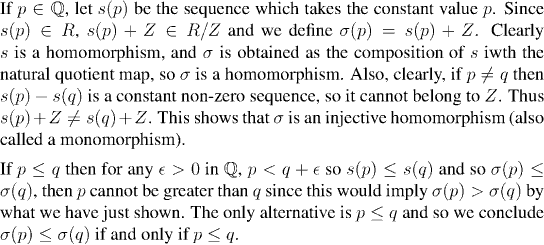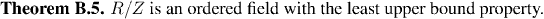Analysis WebNotes
arrow_back arrow_forward

# Class Contents

In Chapter 2, we introduced the real numbers as an ordered field with the least upper bound property. Everything that we did subsequently with R has been a consequence of this description. Yet there are two serious flaws with it, which it is our job to patch here: existence, and uniqueness.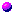Existence It's all very fine to say that R is a field with certain properties, but that begs the question whether any such field exists. After all, one might build up a beautiful theory around a field of six elements, but it would be no good since no such field exists.

Question: Can you prove it?Uniqueness Suppose there were many ordered fields with the least upper bound property. Your "real number system" might not be the same as mine. Might there be useful theorems that held for one ordered field with the least upper bound property that don't hold for others? Which would be the "right" one?

Fortunately, as we asserted in Theorem 2.2, there is exactly one such field. Our job here is to prove Theorem 2.2.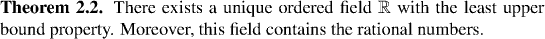The first thing we will do is construct an ordered field with the least upper bound property. There are several ways of doing this but (as we'd expect, since we're planning on proving uniqueness) they all amount to the same thing.
The basic building blocks for the real numbers will be the rational numbers. Notice that the two concepts: convergence to zero, and Cauchy sequence, can be defined without recourse to the real number system: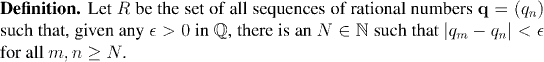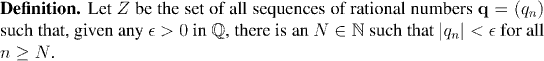Let's think about elements of R for a second. These are sequences of rationals which are "really" Cauchy sequences in R (jumping ahead of ourselves for a minute). So "really" they converge in R, sometimes to rationals sometimes to irrationals.

What's more, every real number has some sequence of rationals converging to it (by the density of the rationals) and so corresponds to some sequences in R.

Of course a given real will correspond to many different sequences in R; any two sequences in R with the same limit in R correspond to the same real. So how can we tell that two sequences in R have the same limit "really" in R, without getting ahead of ourselves and using the real number system?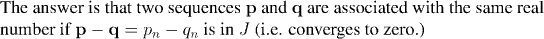Thus the set we get by identifying all sequences which differ by something in J, should be in one to one correspondance with the "real" R. The mathematical object that does this will be the quotient R/J.

Now let's try to put this intuitive argument onto firmer footing.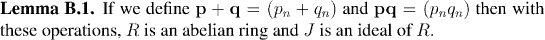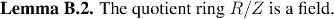We want to show that R/Z is an ordered field with the least upper bound property. Unfortunately, defining the order is a little tricky. Remember that we are thinking of the Cauchy sequences in R as associated with the real numbers which "really" they converge to.

In this vein, how do we tell whether the limit of (p_n) is smaller than the limit of (q_n), without evaluating the limit? Well you can tell that lim pn & lt lim qn by means of the test in the following definition: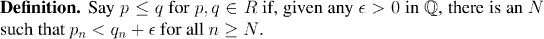Now of course the field we are interested in is R/Z, and our ordering is on R, so we need to carry this over to our ordering on R/Z: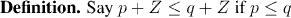Remark:
There is a problem with this definition: it appears to depend of the representatives, p and q, of the classes, p+Z and q+Z, and not just on the classes themselves. We shall show that in fact the definition is independent of the choice of representatives in the next lemma: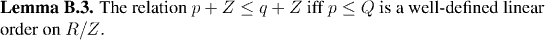Recall from Chapter 2 that there is more to being an ordered field than being a field, together with a linear ordering. The linear ordering must satisfy certain algebraic relationships.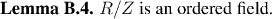Now we must show that R/Z has the least upper bound property. It will be helpful to have a close connection between Q and a subset of R/Z. In the discussion of the next few paragraphs, we develop this: